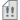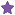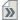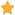###Author Topic: Script for calculating the effective mass in semi-conductors.  (Read 48775 times)

0 Members and 1 Guest are viewing this topic.

####Nordland

• QuantumATK Staff
• Supreme QuantumATK Wizard
•• Posts: 812
• Reputation: 18##### Script for calculating the effective mass in semi-conductors.
« on: February 17, 2009, 00:14 »
If you want to calculate the the effective mass of the electrons and holes in a semi-conductor,
I have created a small script for helping your in doing so. I have attached it to this post.

The script has been updated in a new improved version for support of anisotropic and/or indirect band gap semi-conductors.
And I have modified the tutorial to be on Si, since I could find experimental value of this material.
( However it should be noted, that one must manually enter k-points around which it should evaluate the effective mass,
and if the minimum is present at several places in the brillouin zone, the constant MC and MH must be adjusted represent this.
For instance in Si the lowest point of the conducting band is located 6 places in the brillouin zone, and hence MC must be set to 6)

Simple perform a calculation, and store the results in a NetCDF file,
and edit the script effective_mass.py to point at this netcdf file, and the run it as normal:
atk effective_mass.py

It will produced the following output ( based on a quick Si calculation i maded):
Quote
----------------------------------------------------------------------
# Electron effective mass
# ---> E                     = 0.238248813366
# ---> Conductivity          = 0.25471464737 me
# ---> Density of states     = 1.17006025908 me
# ---> Longitudinal          = 1.3419675337 me
# ---> Transverse (1)        = 0.165647613285 me
# ---> Transverse (2)        = 0.200168158579 me
----------------------------------------------------------------------
# Hole effective mass (1)
# ---> E                     = -0.23358258646
# ---> Conductivity          = 0.641102831335 me
# ---> Density of states     = 0.641102831335 me
# ---> Longitudinal          = 0.641102831305 me
# ---> Transverse (1)        = 0.64110283176 me
# ---> Transverse (2)        = 0.64110283094 me
----------------------------------------------------------------------
# Hole effective mass (2)
# ---> E                     = -0.233582586849
# ---> Conductivity          = 0.64110283086 me
# ---> Density of states     = 0.64110283086 me
# ---> Longitudinal          = 0.64110283089 me
# ---> Transverse (1)        = 0.641102830435 me
# ---> Transverse (2)        = 0.641102831255 me
----------------------------------------------------------------------
# Hole effective mass (3)
# ---> E                     = -0.233582587149
# ---> Conductivity          = 0.0926545669658 me
# ---> Density of states     = 0.0926545669658 me
# ---> Longitudinal          = 0.0926545669658 me
# ---> Transverse (1)        = 0.0926545669658 me
# ---> Transverse (2)        = 0.0926545669658 me
----------------------------------------------------------------------

Silicon has density of states effective mass of 1.08, conductivity effective mass of 0.26, and average hole mass of 0.56,
and I have collected the numbers in a small table below, and I think the agreement is pretty good.

 Effective Mass Experimental Calculation Error Density of States 1.08 1.17 0.09 Conductivity 0.26 0.254 0.006 Hole (average) 0.56 0.46 0.1
« Last Edit: March 14, 2009, 23:09 by Nordland »

####Roc

• QuantumATK Guru
•• Posts: 104
• Reputation: 0##### Re: Script for calculating the effective mass in semi-conductors.
« Reply #1 on: February 23, 2009, 09:41 »
Dear Nordland ，

When I run these scripts  " znse.py and effective_mass.py" , I get the slight different result from yours. as follows,
----------------------------------------------------------------------
# Electron effective mass    = 0.174335561971 me
----------------------------------------------------------------------
# Hole effective mass (1)    = 2.32687348618 me
# Hole effective mass (2)    = 2.11284900262 me
# Hole effective mass (3)    = 0.155049857542 me
----------------------------------------------------------------------
# Average hole effectiv mass = 1.53159078212 me
----------------------------------------------------------------------

what's the reason for that?  (I run the scripts by the version of  2008,10)

Another, whether does it get different results for one same script by  different version like 2008,02 or 2008,10

thanks.

« Last Edit: February 23, 2009, 09:47 by anyipeng »

####Nordland

• QuantumATK Staff
• Supreme QuantumATK Wizard
•• Posts: 812
• Reputation: 18##### Re: Script for calculating the effective mass in semi-conductors.
« Reply #2 on: February 23, 2009, 11:45 »
The difference is very small, and when it is this order it can come from many things, however it is not anything to be worried about.

The platform, windows/linux, and the computer type (32bit/64bit), and many other factors can introduced
these very small deviations, when comparing results perform on machine compared to another.

I have run my example using ATK 2008.10, but I have not observed (and is not aware of ) that should change ATK 2008.02 from ATK 2008.10
in terms of results, when it comes to standard DFT.

####rosen

• Heavy QuantumATK user
•• Posts: 25
• Reputation: 0##### Re: Script for calculating the effective mass in semi-conductors.
« Reply #3 on: March 6, 2009, 04:45 »
Thank you Nordland, but the script seems can only calculated the effectivemass in the G point, if there is an indirect bandgap material, it is improper to use this script, I have revised some of the code in this script to do calculate the effectivemass in an indirect bandgap material, but the result is no so satisfied:
Direct Band Gap =  6.97405383307 eV
Indirect Band Gap = 6.69934035399 eV
----------------------------------------------------------------------
# Electron effective mass    = 0.0219170891857 me
----------------------------------------------------------------------
# Hole effective mass (1)    = 0.069356447541 me
# Hole effective mass (2)    = 0.0345840434497 me
# Hole effective mass (3)    = -2.93394832023 me
----------------------------------------------------------------------
# Average hole effectiv mass = -0.943335943081 me
----------------------------------------------------------------------

(the experimental value of electron effectivemass for alpha SiO2 is 0.5me, and the effective hole mass is 0.38m)

is there anything wrong with my revised script?

####Nordland

• QuantumATK Staff
• Supreme QuantumATK Wizard
•• Posts: 812
• Reputation: 18##### Re: Script for calculating the effective mass in semi-conductors.
« Reply #4 on: March 6, 2009, 12:42 »
The script has a variable callled kpoint, it is set to Gamma now, but can be set to anything, however the script will calculate the effective mass centered around this point. This means for indirect bandgap semiconductors, you will get bad results as the effective mass of the electrons and holes have to be calculated a different k-points, however if you can wait a couple of hours I will try to generalize it to handle indirect semi-conductors correctly.

####rosen

• Heavy QuantumATK user
•• Posts: 25
• Reputation: 0##### Re: Script for calculating the effective mass in semi-conductors.
« Reply #5 on: March 6, 2009, 14:31 »
if you can wait a couple of hours I will try to generalize it to handle indirect semi-conductors correctly.####Nordland

• QuantumATK Staff
• Supreme QuantumATK Wizard
•• Posts: 812
• Reputation: 18##### Re: Script for calculating the effective mass in semi-conductors.
« Reply #6 on: March 6, 2009, 14:53 »
Progress update:

For Silicon I have perfect results, but for SiO2 I have some issues.....- trying to resolve this before posting........

####rosen

• Heavy QuantumATK user
•• Posts: 25
• Reputation: 0##### Re: Script for calculating the effective mass in semi-conductors.
« Reply #7 on: March 12, 2009, 15:11 »
Is there any Progress?

####Nordland

• QuantumATK Staff
• Supreme QuantumATK Wizard
•• Posts: 812
• Reputation: 18##### Re: Script for calculating the effective mass in semi-conductors.
« Reply #8 on: March 12, 2009, 21:57 »
Well, yes- but the results for SiO2 is not very good - perhaps it requires some parameter tweaking.

####Nordland

• QuantumATK Staff
• Supreme QuantumATK Wizard
•• Posts: 812
• Reputation: 18##### Re: Script for calculating the effective mass in semi-conductors.
« Reply #9 on: March 14, 2009, 23:10 »
Rosen, I have reposted the first post with a new script and a new example.

I have found out that it is needed to make sure that the fermi level is very well converged in order to get good effective masses in terms of kpoints.
I use 15,15,15 and it gives good results.

####rosen

• Heavy QuantumATK user
•• Posts: 25
• Reputation: 0##### Re: Script for calculating the effective mass in semi-conductors.
« Reply #10 on: March 15, 2009, 05:18 »
Thank Nordland, I will try to do it in SiO2

####rosen

• Heavy QuantumATK user
•• Posts: 25
• Reputation: 0##### Re: Script for calculating the effective mass in semi-conductors.
« Reply #11 on: March 18, 2009, 08:58 »
in the effective mass calculation, what is the physical meaning of the Conductivity  effectivemass and the Density of states   effectivemass?
Quote
Code
``````print '# Electron effective mass'
print '# ---> E                     = %10s'%(b[step])
print '# ---> Conductivity          = %10s'%(3.0 * (abs((1/ml._value()) + 1/mt1._value() + 1/mt2._value()))**(-1) * me)
print '# ---> Density of states     = %10s'%((MC)**(2.0/3.0) * (ml._value()*mt1._value()*mt2._value())**(1.0/3.0) * me)``````
in wikipedia, the effectivemass is given for one definition. http://en.wikipedia.org/wiki/Effective_mass_(solid-state_physics) besides that, I have do a calculation of the Si with (15, 15, 15) mesh, the band structure (see attach) didn't have 6 lowest point of the conducting band in the brillouin zone?

####Nordland

• QuantumATK Staff
• Supreme QuantumATK Wizard
•• Posts: 812
• Reputation: 18##### Re: Script for calculating the effective mass in semi-conductors.
« Reply #12 on: March 18, 2009, 10:53 »
Hey Rosen
Instead of me trying to explain the physical meaning, I have found two good references, the first
one is on the density of states effective mass. This is the most common printed in papers,
however for conductivity is more convinent to use the conductivity effective mass.

Paper with a note on conductivity effective mass.
Paper on the density of states effective mass

The lowest point in your bandstructure is located at (X,0,X), where X is rougly 0.42156, however there the following six points (0,X,X), ( X,0,X), (X,X,0), (0,-X,-X), (-X, 0, -X), (0, -X, -X) are equivalent in FCC, and hence MC becomes 6 for this point.

I hope this clarifies my ramblings« Last Edit: March 18, 2009, 10:58 by Nordland »

####rosen

• Heavy QuantumATK user
•• Posts: 25
• Reputation: 0##### Re: Script for calculating the effective mass in semi-conductors.
« Reply #13 on: March 26, 2009, 03:48 »
Hi Nordland I have tested your script with the BaTiO3, this model have 5 atoms. the calculated bandstruture is attatched below. for the cubic phase, both the conductiviey and DOS electron effectivemass gave 4.57579367772 me, but the experimental value for this phase is about 0.5 me. and for the tetragonal phase, the # ---> Conductivity          = 0.487857138832 me # ---> Density of states     = 0.805148177393 me I have two question: firstly, from the bandstructure, the curvature at the CBM in G (0. 0. .0.) is almost the same, why the electron effectivemass have so large difference. secondly, in the script, the experssion for the DOS effectivemass is
Code
``````print '# ---> Density of states     = %10s'%((MH)**(2.0/3.0) * (abs(ml._value()*mt1._value()*mt2._value()))**(1.0/3.0) * me)
``````
,  but according to the paper you give us Paper with a note on conductivity effective mass.http://mems.caltech.edu/courses/EE40%20Web%20Files/Supplements/01_Effective_Mass.pdf, it should be
Code
`````` Density of states     = %10s'%((MH)**(3.0/2.0) * (abs(ml._value()*mt1._value()*mt2._value()))**(1.0/3.0) * me)
``````
, it will affect the result of Si, but has no influence on the BaTiO3 here.
« Last Edit: March 26, 2009, 06:00 by rosen »

####Nordland

• QuantumATK Staff
• Supreme QuantumATK Wizard
•• Posts: 812
• Reputation: 18##### Re: Script for calculating the effective mass in semi-conductors.
« Reply #14 on: March 26, 2009, 07:28 »
Hey Rosen.

Thanks for testing my script and correcting my bugs- I will upload new version soon.

I think I know why there is a difference between the two phases - it is properly an artifact, but i think that I can explain the two effective mass from the bandstructure.

In the cubic phase at the Gamma point ( to the left most of your bandstructure ) there are two bands - one which is relative heavy perhaps roughly 4.0 - 7.0 ( the very flat one ) and one that is order of 0.5 - 1.0 ( the normal shaped one ).
The bands appears to be very close and perhaps degenerated at the gamma point, and perhaps there we end up with the wrong effective mass.

However in the tetragonal phase there is a spliting between these two bands, and then we don't have this issue of these two bands mixing in together.

I will try rerunning your calculation with the aim of finding out the differences